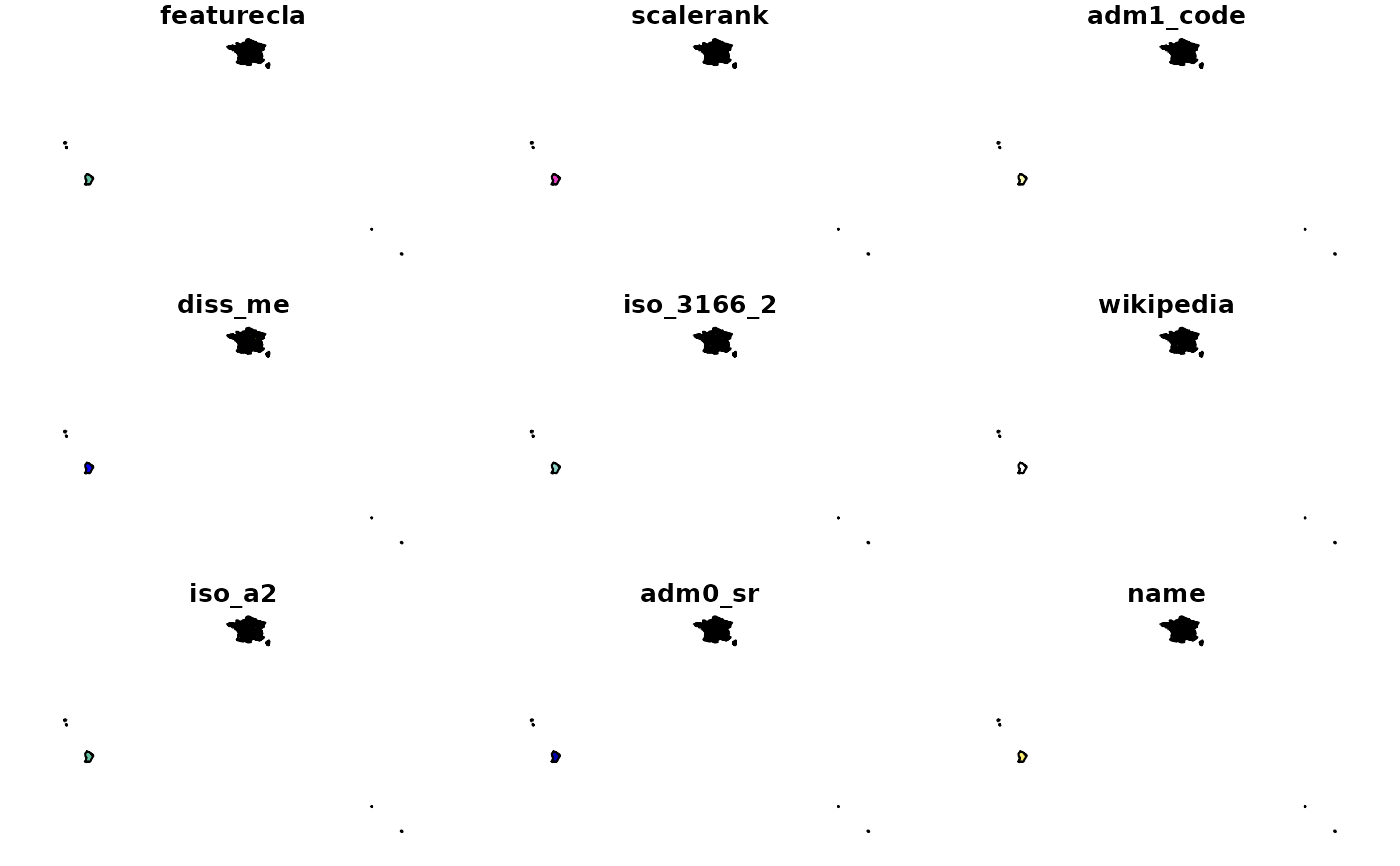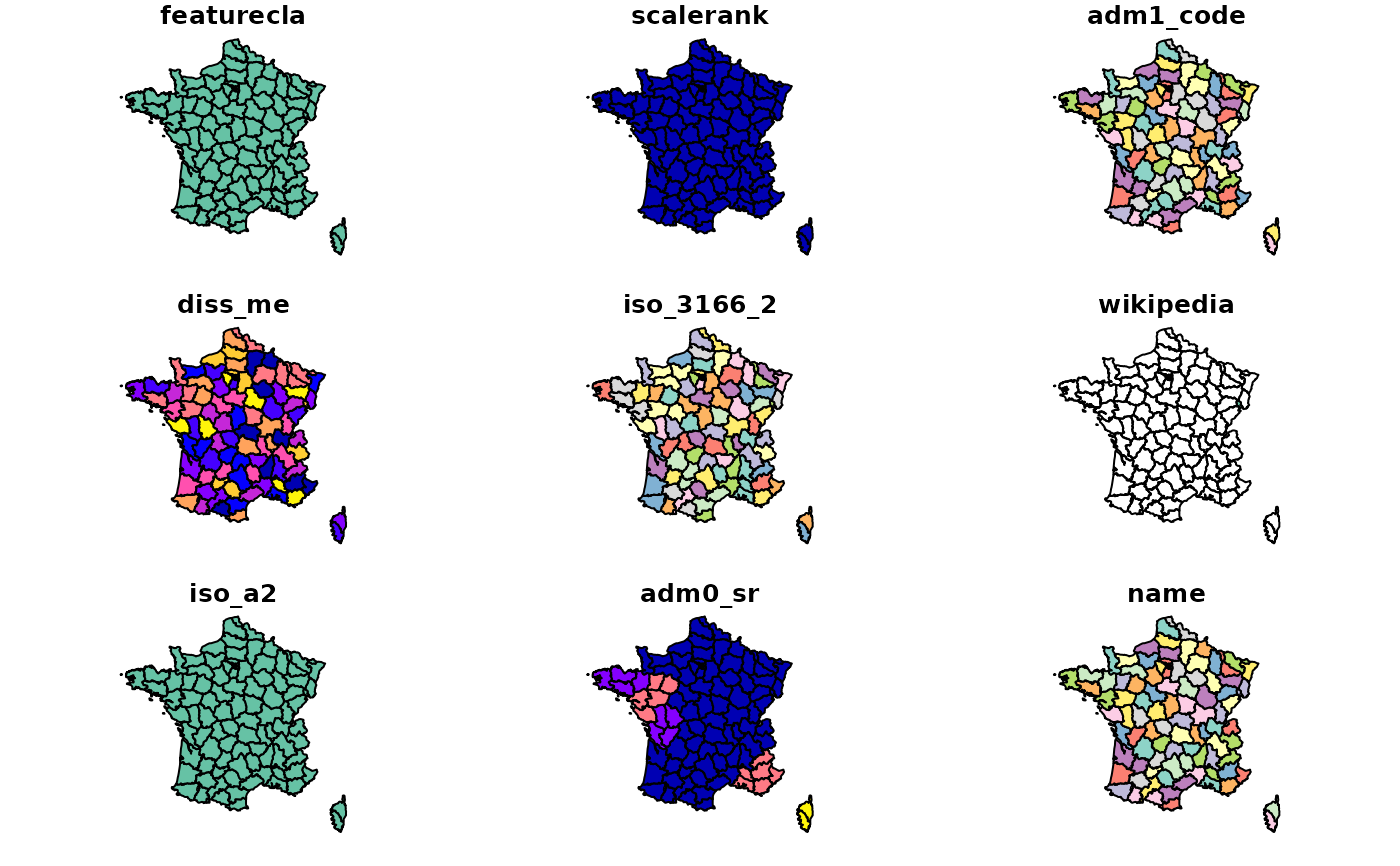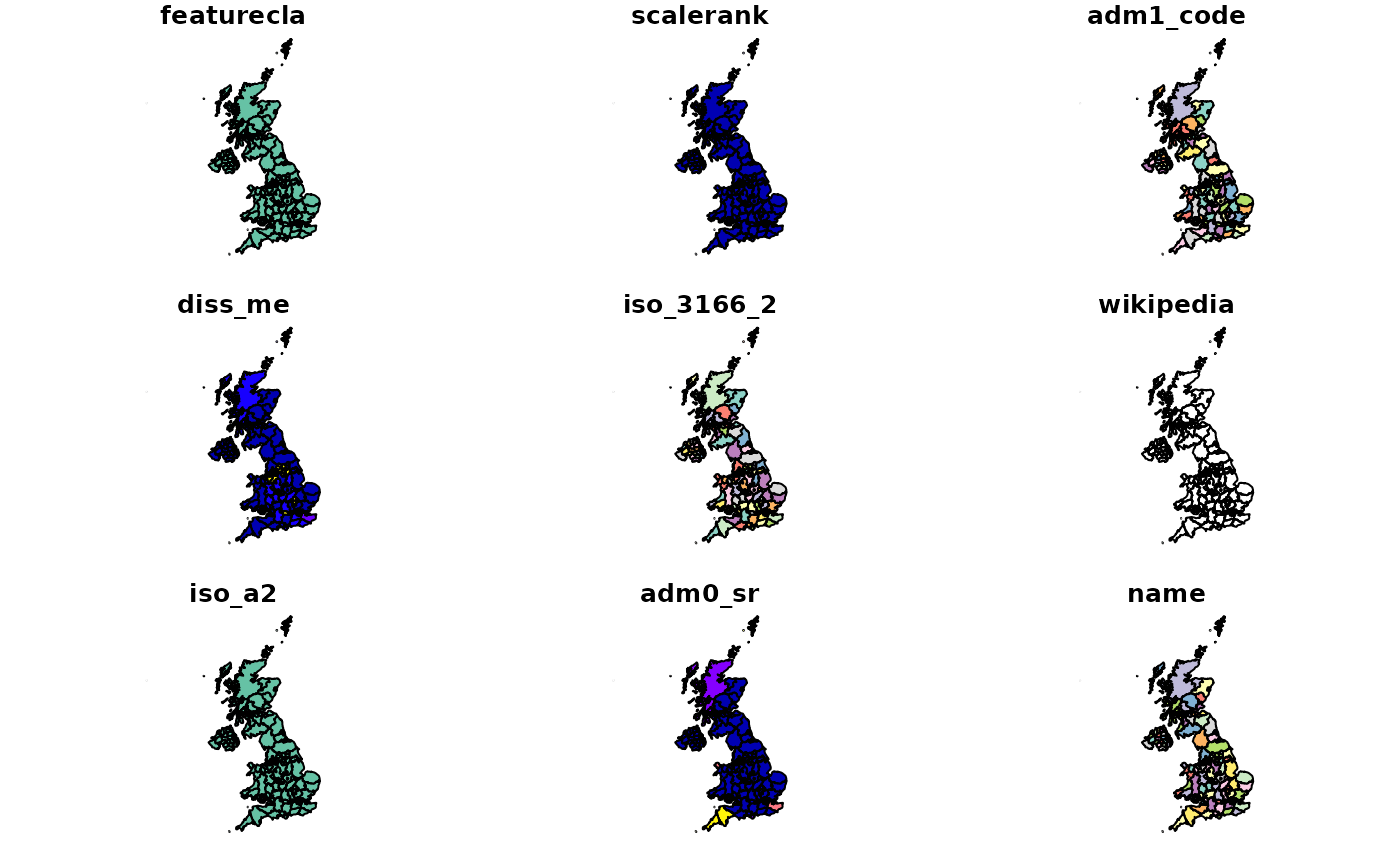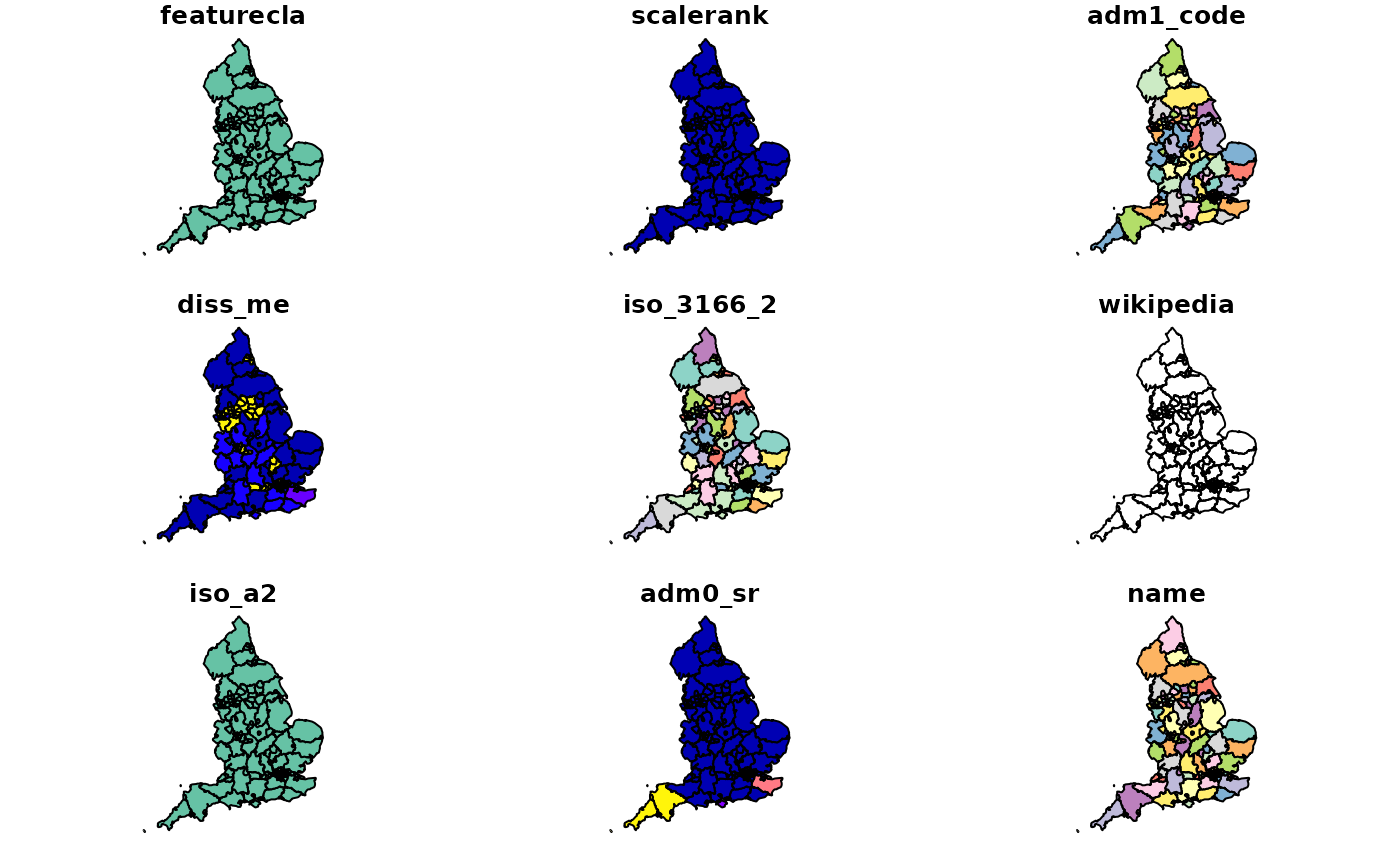returns state polygons (administrative level 1) for specified countries

## Usage

``````ne_states(
country = NULL,
geounit = NULL,
iso_a2 = NULL,
spdf = NULL,
returnclass = c("sp", "sf")
)``````

## Arguments

country

a character vector of country names.

geounit

a character vector of geounit names.

iso_a2

a character vector of iso_a2 country codes

spdf

an optional alternative states map

returnclass

'sp' default or 'sf' for Simple Features

## Value

`SpatialPolygonsDataFrame` or `sf`

## Examples

``````
# comparing using country and geounit to filter
if (requireNamespace("rnaturalearthhires")) {
spdf_france_country <- ne_states(country = 'france')
spdf_france_geounit <- ne_states(geounit = 'france')
if (require(sp)) {
plot(spdf_france_country)
plot(spdf_france_geounit)

plot(ne_states(country = 'united kingdom'))
plot(ne_states(geounit = 'england'))
}
}